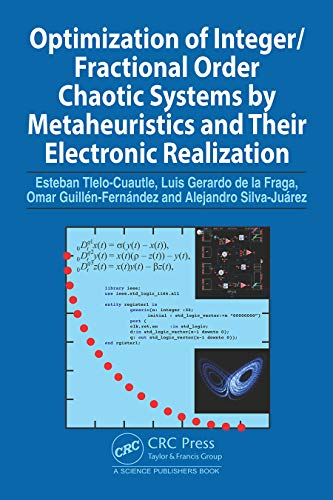# Optimization of Integer/Fractional Order Chaotic Systems by Metaheuristics and their Electronic Realization

• Length: 266 pages
• Edition: 1
• Publisher:
• Publication Date: 2021-05-10
• ISBN-10: 0367486687
• ISBN-13: 9780367486686
Description

Mathematicians have devised different chaotic systems that are modeled by integer or fractional-order differential equations, and whose mathematical models can generate chaos or hyperchaos. The numerical methods to simulate those integer and fractional-order chaotic systems are quite different and their exactness is responsible in the evaluation of characteristics like Lyapunov exponents, Kaplan-Yorke dimension, and entropy. One challenge is estimating the step-size to run a numerical method. It can be done analyzing the eigenvalues of self-excited attractors, while for hidden attractors it is difficult to evaluate the equilibrium points that are required to formulate the Jacobian matrices. Time simulation of fractional-order chaotic oscillators also requires estimating a memory length to achieve exact results, and it is associated to memories in hardware design. In this manner, simulating chaotic/hyperchaotic oscillators of integer/fractional-order and with self-excited/hidden attractors is quite important to evaluate their Lyapunov exponents, Kaplan-Yorke dimension and entropy. Further, to improve the dynamics of the oscillators, their main characteristics can be optimized applying metaheuristics, which basically consists of varying the values of the coefficients of a mathematical model. The optimized models can then be implemented using commercially available amplifiers, field-programmable analog arrays (FPAA), field-programmable gate arrays (FPGA), microcontrollers, graphic processing units, and even using nanometer technology of integrated circuits.

The book describes the application of different numerical methods to simulate integer/fractional-order chaotic systems. These methods are used within optimization loops to maximize positive Lyapunov exponents, Kaplan-Yorke dimension, and entropy. Single and multi-objective optimization approaches applying metaheuristics are described, as well as their tuning techniques to generate feasible solutions that are suitable for electronic implementation. The book details several applications of chaotic oscillators such as in random bit/number generators, cryptography, secure communications, robotics, and Internet of Things.

To access the link, solve the captcha.